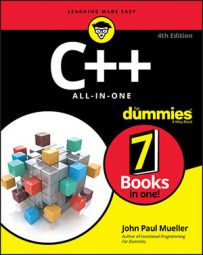##### C++ All-in-One For DummiesMost containers let you perform random access of data they contain. For example, the RandomAccess example shows that you can create an iterator and then add to or subtract from the current offset to obtain values within the container that iterator supports:

```#include <iostream>
#include <vector>
using namespace std;
int main()
{
vector<string> Words;
Words.push_back("Blue");
Words.push_back("Green");
Words.push_back("Teal");
Words.push_back("Brick");
Words.push_back("Purple");
Words.push_back("Brown");
Words.push_back("LightGray");
// Define a random iterator.
vector<string>::iterator Iter = Words.begin();
// Access random points.
Iter += 5;
cout << *Iter << endl;
Iter -= 2;
cout << *Iter << endl;
return 0;
}```

In this case, the vector, Words, contains a list of seven items. The code creates an iterator for Words named Iter. It then adds to or subtracts from the iterator offset and displays the output onscreen. Here is what you see when you run this example:

```Brown
Brick```

Sometimes you need to perform a special task using a random-access iterator. For example, you might want to create a special function to summate the members of vector or just a range of members within vector.

In this case, you must create a specialized function to perform the task as follows because the Standard Library doesn’t include any functions to do it for you, as shown in the RandomAccess2 example:

```#include <iostream>
#include <vector>
using namespace std;
template <class RandomAccessIterator>
{
float Sum = 0;
RandomAccessIterator Index;
// Make sure that the values are in the correct order.
if (begin > end)
{
RandomAccessIterator temp;
temp = begin;
begin = end;
end = temp;
}
for (Index = begin; Index != end; Index++)
Sum += *Index;
return Sum;
}
int main()
{
vector<float> Numbers;
Numbers.push_back(1.0);
Numbers.push_back(2.5);
Numbers.push_back(3.75);
Numbers.push_back(1.26);
Numbers.push_back(9.101);
Numbers.push_back(11.3);
Numbers.push_back(1.52);
// Sum the individual members.
float Sum;
cout << Sum << endl;
cout << Sum << endl;
// Sum a range.
vector<float>::iterator Iter = Numbers.begin();
Iter += 5;
cout << Sum << endl;
return 0;
}```

This example builds on the previous example. You still create vector, Numbers, and fill it with data. However, in this case, you create an output variable, Sum, that contains the summation of the elements contained in Numbers.

AddIt() is a special function that accepts two RandomAccessIterator values as input. These two inputs represent a range within the vector that you want to manipulate in some way. The example simply adds them, but you can perform any task you want. The output is a float that contains the summation.

AddIt() works as you expect. You call it as you would any other function and provide a beginning point and an end point within vector. The first two calls to AddIt sum the entire vector, while the third creates an iterator, changes its offset, and then sums a range within vector. Here is the output from this example:

```30.431
30.431
12.82```

A random-access iterator can go in either direction. In addition, you can work with individual members within the container supplied to iterator. As a result, the functions you create for iterator must be able to work with the inputs in any order. How you handle this requirement depends on the kind of function you create.